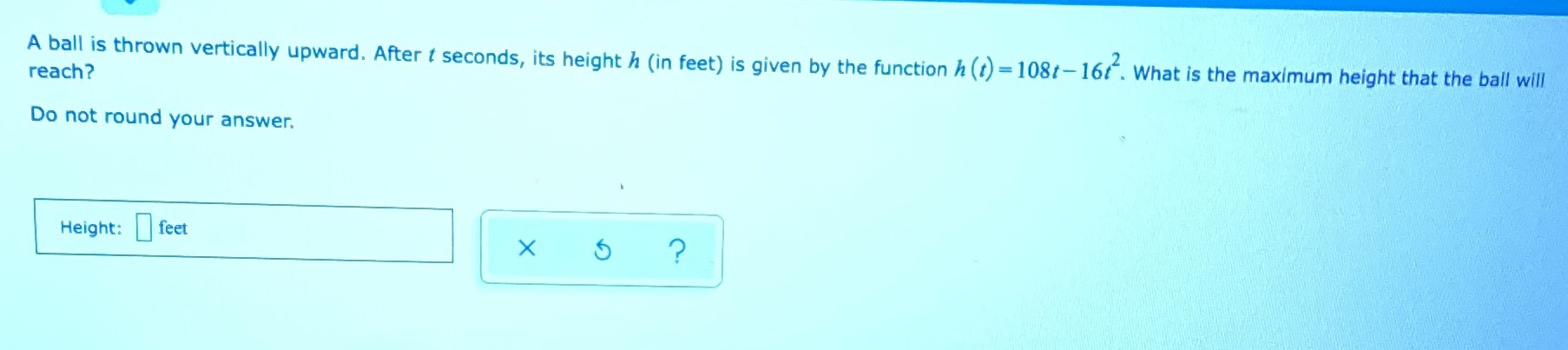### Still have math questions?

Trigonometry
QuestionA ball is thrown vertically upward. After $$t$$ seconds, its height $$h$$ (in feet) is given by the function $$h ( t ) = 108 t - 16 t ^ { 2 }$$ . What is the maximum height that the ball will reach?

Height: $$\square$$ feet
$$\frac{729}{4}$$